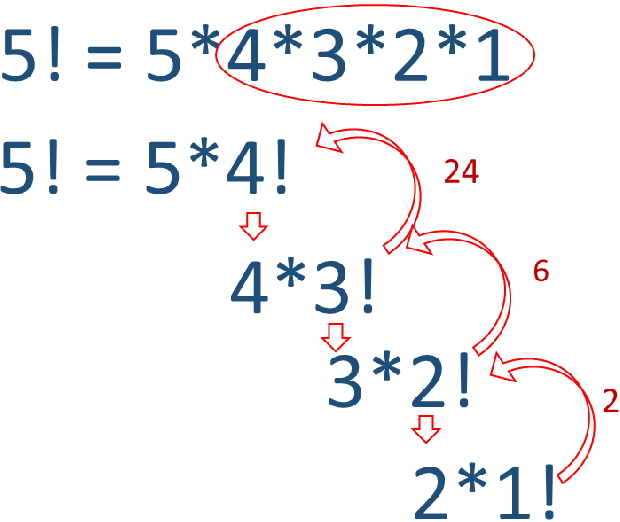# Factorial of a number in Python

Factorial of a number is product of all integers from 1 up to the number ( inclusive ).
Factorial of 5 is 1*2*3*4*5 = 120
Or
n! = 1*2*3….n

Factorial of a number by using loop and by recursive functions in Python

## Factorial of an input number by using loop

We used for loop here
``````a=int(input("Enter a number : "))
b=1
for i in range(1,a+1):
b=b*i
print("Factorial : ", b)``````
Output
``````Enter a number : 6
Factorial :  720``````

## Factorial of a number by using recursive functionBy using recursive function we can call the function itself from within the function.
``````def fact(n):
if n==1:
return 1
else:
result=n* fact(n-1)
#print("Part factorial : ", result)
return result
fact(4)``````
Output
``24``

Subscribe to our YouTube Channel here

## Subscribe

* indicates required
Subscribe to plus2netplus2net.com